# Iterators Containers Bidirectional iterators for list map set

• Slides: 25Iterators & Containers �Bidirectional iterators for: �list, map, set �Random access iterators for: �vector �Input/output/forward iterators for: �iostreams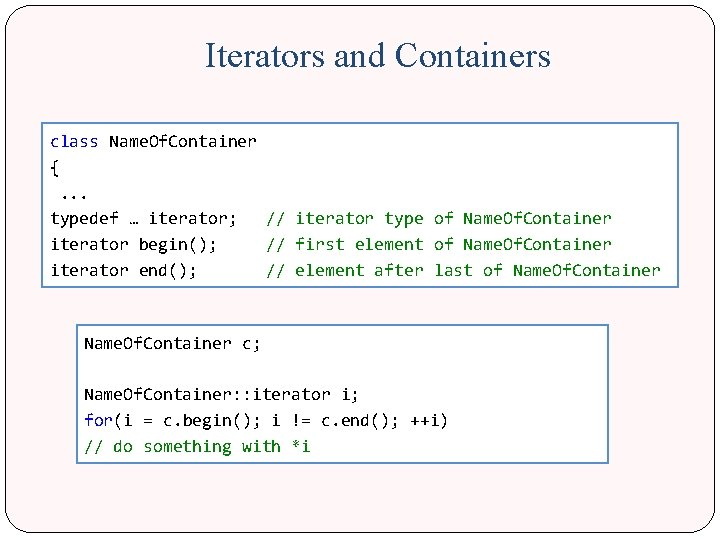Iterators and Containers class Name. Of. Container { . . . typedef … iterator; // iterator type of Name. Of. Container iterator begin(); // first element of Name. Of. Container iterator end(); // element after last of Name. Of. Container c; Name. Of. Container: : iterator i; for(i = c. begin(); i != c. end(); ++i) // do something with *iIterators and Containers �With templates: Name. Of. Container<. . . > c . . . Name. Of. Container<. . . >: : iterator i; for(i= c. begin(); i!=c. end(); ++i) // do something that changes *i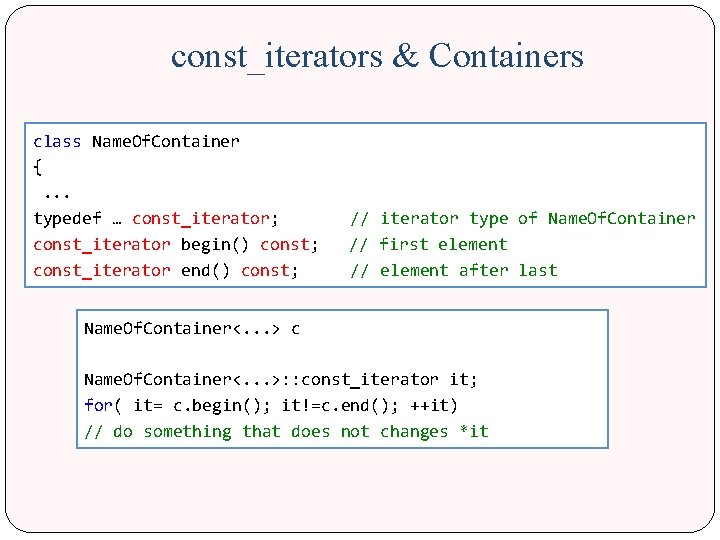const_iterators & Containers class Name. Of. Container { . . . typedef … const_iterator; // iterator type of Name. Of. Container const_iterator begin() const; // first element const_iterator end() const; // element after last Name. Of. Container<. . . > c Name. Of. Container<. . . >: : const_iterator it; for( it= c. begin(); it!=c. end(); ++it) // do something that does not changes *it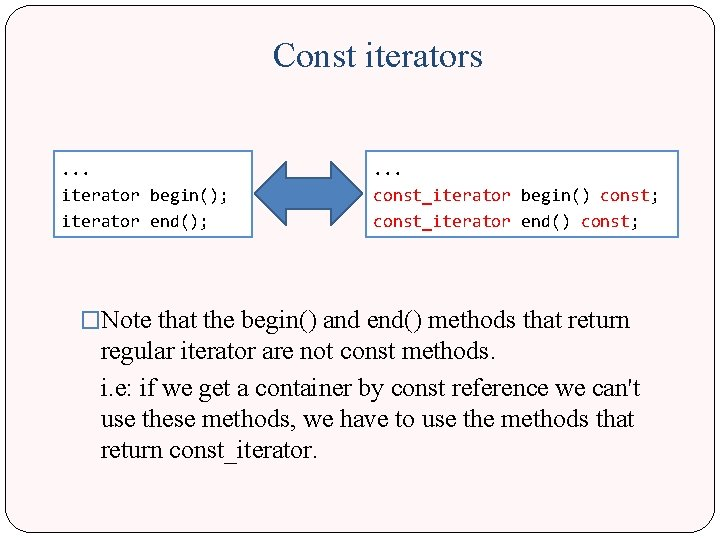Const iterators. . . iterator begin(); iterator end(); . . . const_iterator begin() const; const_iterator end() const; �Note that the begin() and end() methods that return regular iterator are not const methods. i. e: if we get a container by const reference we can't use these methods, we have to use the methods that return const_iterator.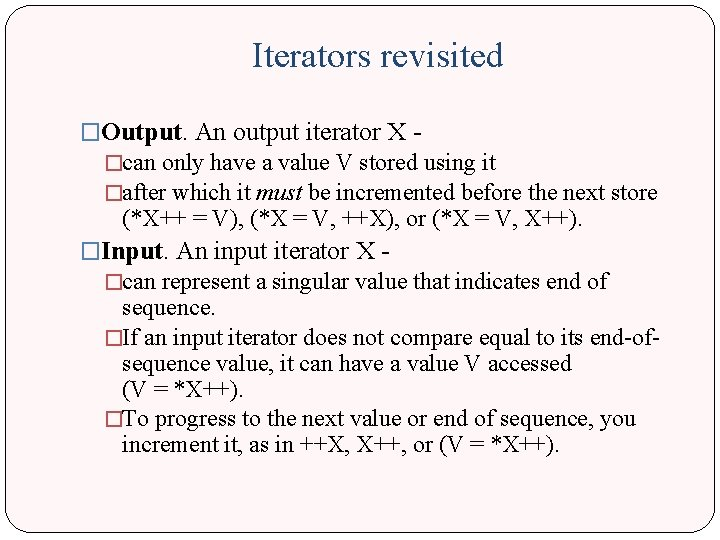Iterators revisited �Output. An output iterator X �can only have a value V stored using it �after which it must be incremented before the next store (*X++ = V), (*X = V, ++X), or (*X = V, X++). �Input. An input iterator X - �can represent a singular value that indicates end of sequence. �If an input iterator does not compare equal to its end-ofsequence value, it can have a value V accessed (V = *X++). �To progress to the next value or end of sequence, you increment it, as in ++X, X++, or (V = *X++).Iterators revisited � Forward. A forward iterator X - �can take the place of an output iterator for writing or an input iterator for reading. �You can read (through V = *X) what you just wrote (through *X = V) through a forward iterator. �You can also make multiple copies of a forward iterator, each of which can be dereferenced and incremented independently. � Bidirectional. A bidirectional iterator X - STL Containers have �can take the place of a forward iterator. at least this type �You can, however, also decrement a bidirectional iterator, as in --X, X--, or (V = *X--). � Random access. A random-access iterator X - �can take the place of a bidirectional iterator. �You can also perform much the same integer arithmetic on a random -access iterator that you can on an object pointer. For N (integer), you can write x[N], x + N, x - N, and N + X.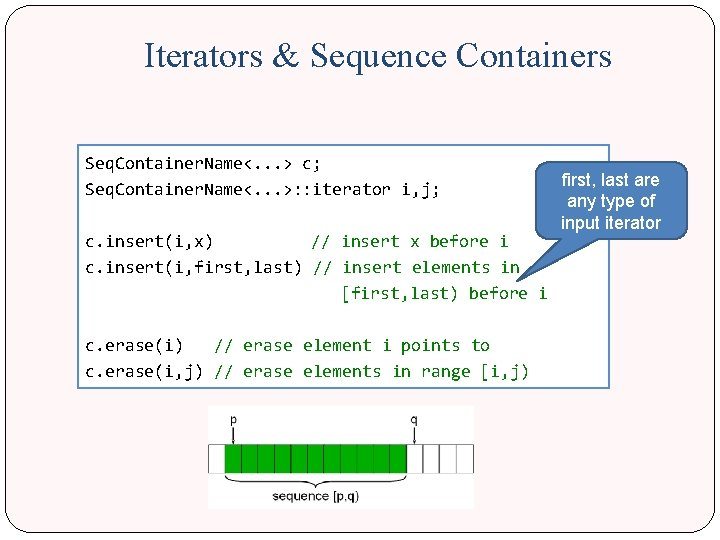Iterators & Sequence Containers Seq. Container. Name<. . . > c; first, last are Seq. Container. Name<. . . >: : iterator i, j; any type of input iterator c. insert(i, x) // insert x before i c. insert(i, first, last) // insert elements in [first, last) before i c. erase(i) // erase element i points to c. erase(i, j) // erase elements in range [i, j)Iterators & other Containers �insert and erase has the same ideas for different containers, except they keep the invariants of the specific container. �For example, a Sorted Associative Container will remain sorted after insertions and erases.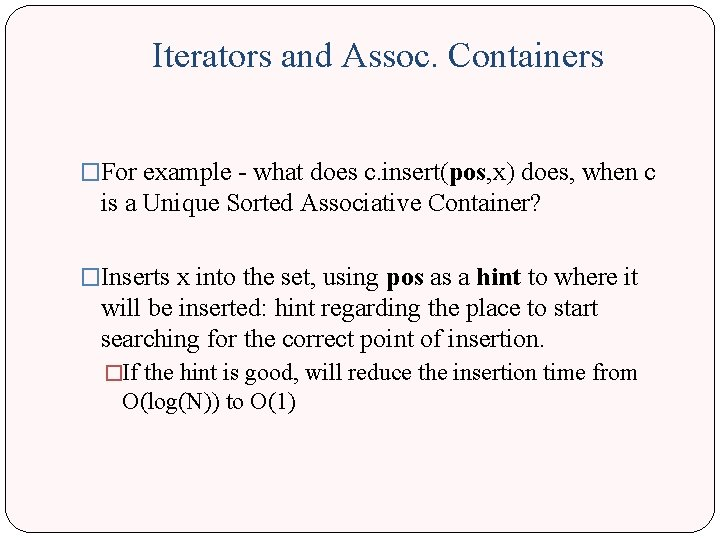Iterators and Assoc. Containers �For example - what does c. insert(pos, x) does, when c is a Unique Sorted Associative Container? �Inserts x into the set, using pos as a hint to where it will be inserted: hint regarding the place to start searching for the correct point of insertion. �If the hint is good, will reduce the insertion time from O(log(N)) to O(1)Iterators and Assoc. Containers Additional set of operations: � iterator c: : find(key_type const& key) Returns iterator to first element with key � iterator c: : lower_bound(key_type const& key) Returns iterator to first element greater or equal to key � iterator c: : upper_bound(key_type const& key) Returns iterator to first element greater than key � end() if not found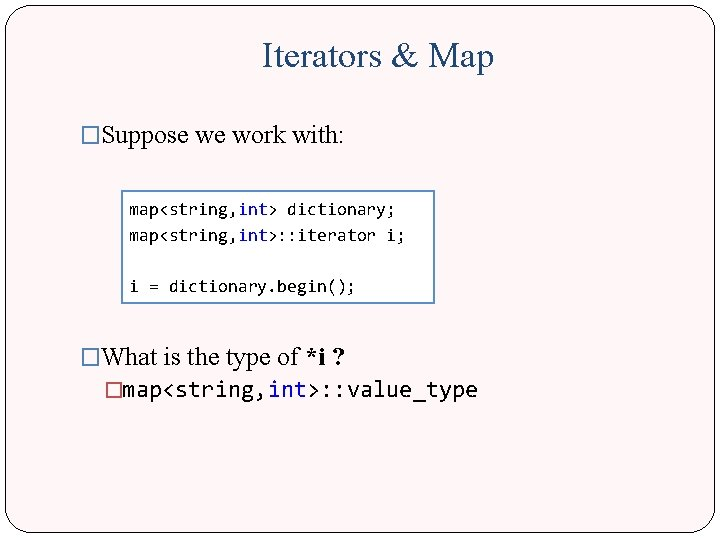Iterators & Map �Suppose we work with: map<string, int> dictionary; map<string, int>: : iterator i; i = dictionary. begin(); �What is the type of *i ? �map<string, int>: : value_typeIterators & Map �Ok – but what is it? map<Key. Type, Value. Type> keeps pairs: Key. Type key – “key” of entry Value. Type value – “value” of entry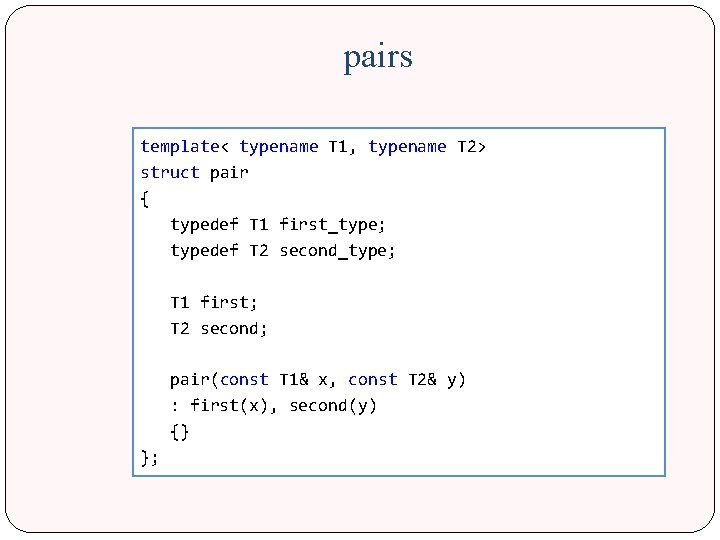pairs template< typename T 1, typename T 2> struct pair { typedef T 1 first_type; typedef T 2 second_type; T 1 first; T 2 second; pair(const T 1& x, const T 2& y) : first(x), second(y) {} };Map value_type template<typename Key, typename T, typename Cmp = less<Key>> class map { public: typedef pair<const Key, T> value_type; typedef Key key_type; typedef T mapped_type; typedef Cmp key_compare; };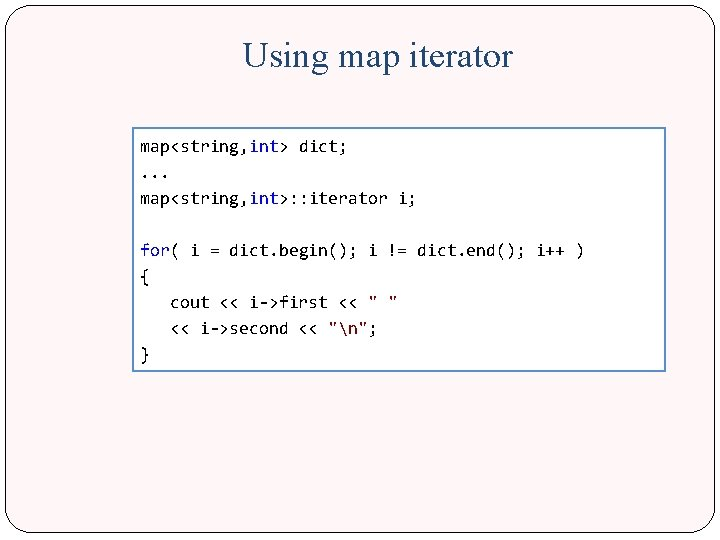Using map iterator map<string, int> dict; . . . map<string, int>: : iterator i; for( i = dict. begin(); i != dict. end(); i++ ) { cout << i->first << " " << i->second << "n"; }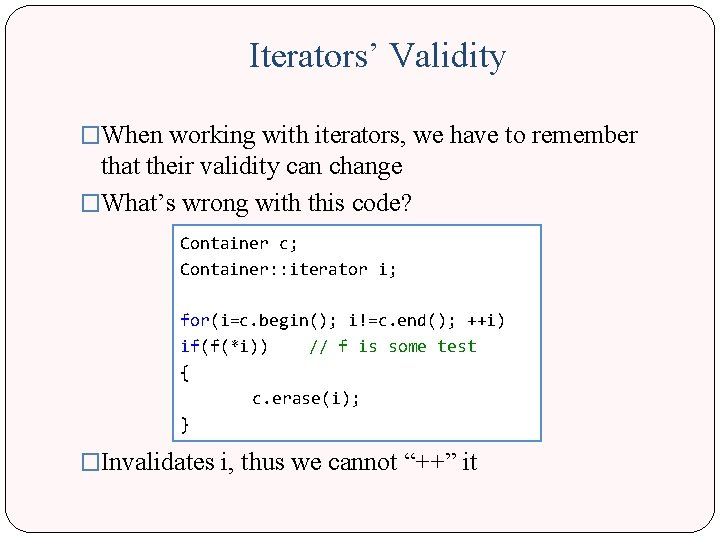Iterators’ Validity �When working with iterators, we have to remember that their validity can change �What’s wrong with this code? Container c; Container: : iterator i; for(i=c. begin(); i!=c. end(); ++i) if(f(*i)) // f is some test { c. erase(i); } �Invalidates i, thus we cannot “++” itIterators’ Validity Two cases: �list, set, map �i is not a legal iterator �vector �i (might) point to the element after �In either case, this is not what we want…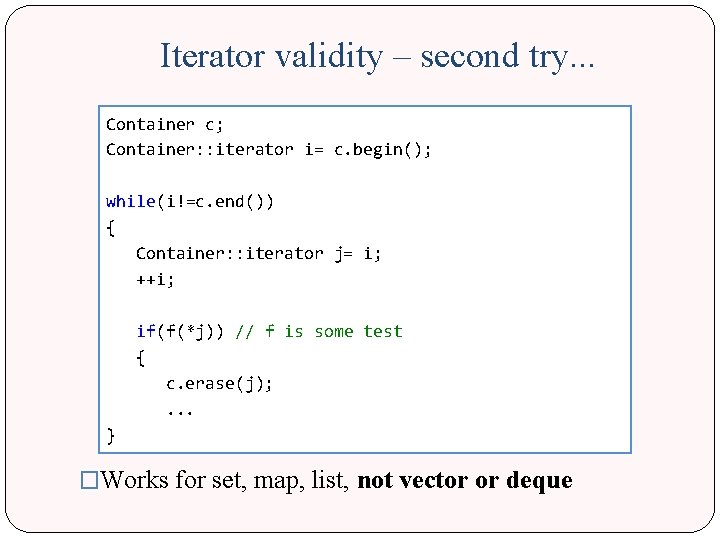Iterator validity – second try. . . Container c; Container: : iterator i= c. begin(); while(i!=c. end()) { Container: : iterator j= i; ++i; if(f(*j)) // f is some test { c. erase(j); . . . } �Works for set, map, list, not vector or dequeHow do you know? ! �Experience �Trial and error ; ) �http: //www. cplus. com/reference/stl/ �msdn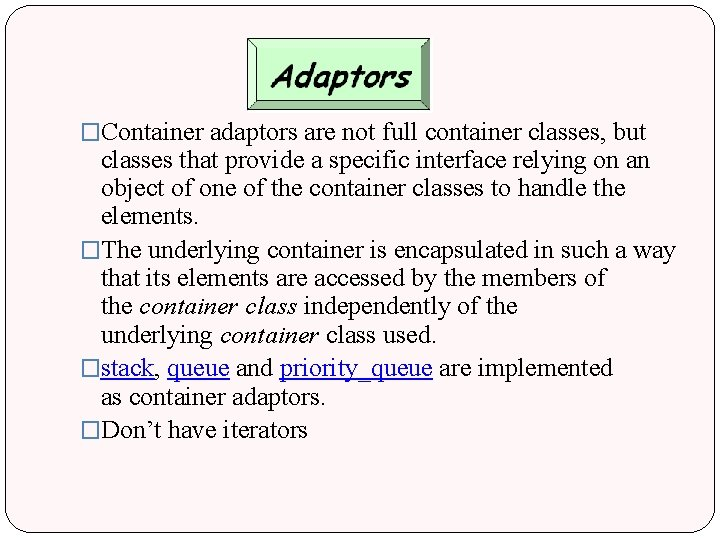�Container adaptors are not full container classes, but classes that provide a specific interface relying on an object of one of the container classes to handle the elements. �The underlying container is encapsulated in such a way that its elements are accessed by the members of the container class independently of the underlying container class used. �stack, queue and priority_queue are implemented as container adaptors. �Don’t have iteratorsStack template <class T, class Container=deque<T>> class stack; �T – stored type �Container - the only requirement is that it supports the following operations �back() �push_back() �pop_back()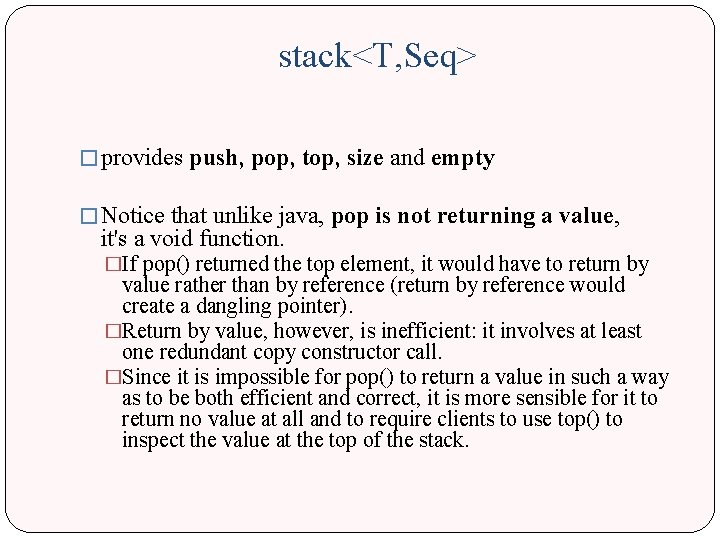stack<T, Seq> � provides push, pop, top, size and empty � Notice that unlike java, pop is not returning a value, it's a void function. �If pop() returned the top element, it would have to return by value rather than by reference (return by reference would create a dangling pointer). �Return by value, however, is inefficient: it involves at least one redundant copy constructor call. �Since it is impossible for pop() to return a value in such a way as to be both efficient and correct, it is more sensible for it to return no value at all and to require clients to use top() to inspect the value at the top of the stack.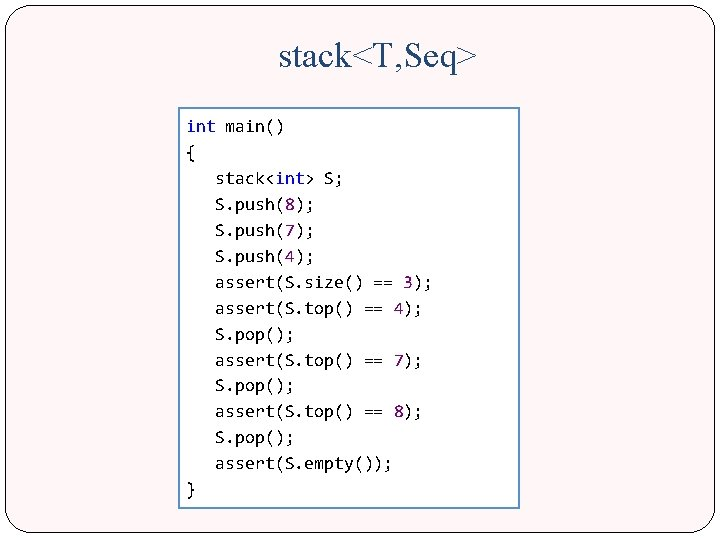stack<T, Seq> int main() { stack<int> S; S. push(8); S. push(7); S. push(4); assert(S. size() == 3); assert(S. top() == 4); S. pop(); assert(S. top() == 7); S. pop(); assert(S. top() == 8); S. pop(); assert(S. empty()); }